U.S. Department of Transportation
1200 New Jersey Avenue, SE
Washington, DC 20590
202-366-4000

Federal Highway Administration Research and Technology
Coordinating, Developing, and Delivering Highway Transportation Innovations

 REPORT This report is an archived publication and may contain dated technical, contact, and link information
 Publication Number:  FHWA-HRT-16-009    Date:  March 2017
 Publication Number: FHWA-HRT-16-009 Date: March 2017

# Using Falling Weight Deflectometer Data With Mechanistic-Empirical Design and Analysis, Volume I: Final Report

## CHAPTER 6. FWD DATA ANALYSIS AND INTERPRETATION—COMPOSITE PAVEMENTS

### Introduction

In this chapter, FWD data analysis and interpretation of HMA/PCC pavements are discussed. Only pavements consisting of an intact PCC pavement overlaid with HMA are considered. Other types of pavements behave more as either a flexible or rigid pavement system; therefore, those methods discussed in chapter 4 or 5 are applicable. For example, if an existing PCC pavement is rubblized prior to overlaying with HMA, the resulting pavement behaves as a flexible pavement. Similarly, if an existing HMA pavement is overlaid with PCC (whitetopping), the resulting pavement is equivalent to a PCC pavement with an HMA base. Although the behavior of an HMA/PCC pavement is dominated by the underlying PCC pavement, the evaluation of FWD data collected on HMA/PCC pavements warrants special considerations because of the compression of the HMA layer that occurs when the load plate impacts the pavement surface.

Asphalt resurfacing is one of the more commonly used methods for PCC pavement rehabilitation. As of 2008, composite pavements comprised almost 23 percent of all pavement types on the National Highway System, most of which were originally PCC pavements.(107) Thus, the evaluation of HMA/PCC pavements is an important topic for State departments of transportation, especially because the life of the composite pavements can be extended even further through the application of timely and effective maintenance and rehabilitation strategies.

FWD testing plays an important role in the evaluation of HMA/PCC pavements. As with other pavement types, deflection testing can be used to evaluate the structural integrity of the underlying PCC slabs, the degree of foundation support, and the LTE across joints and cracks. As with PCC pavements, effective LTE across transverse joints and cracks is important for good performance of composite pavements. Poor LTE across joints and cracks leads to premature development of reflection cracking in the HMA overlay and faster deterioration of the reflected cracks.(108)

The single factor that distinguishes the analysis of FWD testing conducted on composite pavements from that conducted on conventional PCC pavements is the effect of compression of the HMA layer. Backcalculation analyses conducted without considering the effects of the compression that occurs in the HMA layer leads to significant errors.(54) Moreover, compression of the HMA layer also poses a problem in LTE testing for composite pavements. For example, the additional apparent deflection resulting from the compression of the HMA layer leads to lower apparent LTE than actually exists. One way to minimize this effect is to take the loaded-side deflection away from the load plate, as shown in figure 113. A similar approach is used in backcalculation to cope with the effects of the compression in the HMA layer. In this case, the recommended methods involve excluding the deflection under the load plate in the analysis.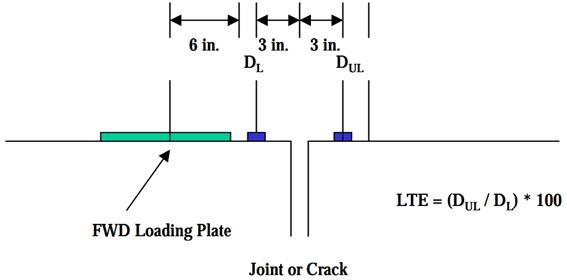1 inch = 25.4 mm.

Figure 113. Diagram. Layout of joint LTE testing on HMA/PCC.(108)

Presented in this chapter are the approaches, available software, and modeling issues for backcalculation of composite pavements, along with recommendations on the use of FWD data with the MEPDG. Note that one way to avoid all complications caused by the presence of the HMA overlay is to remove all HMA before FWD testing, but this may not always be practical or cost effective, depending on the thickness of the overlay. Thus, for planning purposes, the ability to evaluate the FWD data from composite pavements is desirable.

### APPROACHES

Backcalculation for composite pavements can be accomplished using methods similar to those discussed in chapter 5 for rigid pavements. As for bare PCC pavements, the available backcalculation methods include the AREA method and the Best-Fit method.(51) Again, the main factor that complicates backcalculation of composite pavements is the compression that takes place within the HMA layer. Methods have been developed for handling the compression of the HMA layer, and it has been demonstrated that when the compression of the HMA layer is properly handled, both the pavement layer stiffness and the subgrade k-value can be reliably backcalculated from FWD data.

Several researchers have also demonstrated the feasibility of accomplishing backcalculation for composite pavements using ANNs to facilitate the calculation process.(109,110) The principal advantage of the ANN approach is that, based on the results of a limited number of training cases, the pavement response for any combination of input values can be determined almost instantaneously. The training cases are analyzed using the analysis tool that is capable of modeling all effects that are important for the subject pavement type. For example, for composite pavements, an analysis tool that is capable of modeling the compression in the HMA layer would be used. The ANN developed in this way can then be used as the rapid-solution tool to identify the pavement layers and subgrade moduli that best fit the deflection data. Although this is a promising technology, no software is yet available for general use.

### AVAILABLE METHODS

This section presents a description of two methods of backcalculation for composite pavements that are available for general use: the outer-AREA method and the Best-Fit method. While no ready-to-use software is available for either method, they are simple to implement. The outer-AREA method, for example, can be easily implemented in a spreadsheet.

### Outer AREA Method

The AREA method (discussed in chapter 5) is perhaps the most widely used method of backcalculation for rigid pavements. As described in chapter 5, AREA is the normalized area of the deflection basin.(50) Because the sensor deflections are first normalized by the deflection under the load plate (d0) in calculating the AREA, the results of backcalculation by the AREA method are sensitive to the d0 deflection. Therefore, the compression that occurs in the HMA layer is significant and must be taken into account. In one approach, the deflection under the load plate is corrected for the compression of the HMA layer to simulate the deflection profile of plain concrete pavement.(111) It was later found that excellent results can be obtained by performing the backcalculation without the deflection under the load plate (d0) and instead using only the deflections from sensors 305 mm (12 inches) and farther away from the load plate.(54) This approach, which is commonly called the outer-AREA method, was first introduced by Hall et al.(51) McPeak et al. also showed that the outer-AREA method works well in determining the k-value from FWD testing conducted on flexible pavements for the evaluation of PCC overlays of existing HMA pavements.(112)

The calculation procedure for the outer-AREA method is the same as that for the AREA method, except that AREA is calculated without the d0 deflection, with the deflections normalized by the d12 deflection. The outer-AREA for seven sensors at uniform 305-mm (12-inch) spacings is determined as shown in figure 114.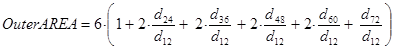Figure 114. Equation. Outer-AREA for seven-sensor configuration.

Where:

dr = FWD deflections at distance r from the center of the load plate, mm (inches).

Following the approach introduced by Hall et al., McPeak and Khazanovich derived the relationship (shown in figure 115) between the radius of relative stiffness (ℓ) and the outer-AREA for the seven-sensor configuration.(51,54)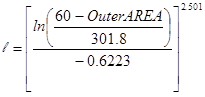Figure 115. Equation. Relationship between the radius of relative stiffness (ℓ) and the outer-AREA for the seven-sensor configuration.(51,54)

Once the radius of relative stiffness is determined, the k-value can be determined from any of the measured deflections using the relationship shown in figure 116.(51)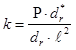Figure 116. Equation. k-value determination.(51)

Where:

k = Modulus of subgrade reaction, MPa/mm, (lbf/inch2/inch).
P = Applied load, N (lbf).
dr* = Nondimensional deflection coefficient for deflection at radial distance r from the center of the load plate.
dr = Measured deflection at radial distance r from the load, mm (inches).
ℓ = Radius of relative stiffness, mm (inches).

The nondimensional deflection coefficient for deflection at radial distance r from the center of the load plate is given by the figure 117 equation.(51)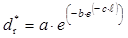Figure 117. Equation. Nondimensional deflection coefficient for deflection.(51)

Where:

a, b, c = Regression coefficients (see table 12).
ℓ = Radius of relative stiffness, mm (inches).

Table 12. Coefficients for nondimensional deflection coefficients.(51)

inches

a

b

c

12

0.12188

0.79432

0.07074

24

0.11634

2.06115

0.06775

36

0.10960

3.62187

0.06568

48

0.10241

5.41549

0.06402

60

0.09521

7.41241

0.06255

72

0.08822

9.59399

0.06118

1 inch = 25.4 mm.
Note: Coefficients are for use with U.S. customary units.

Once the radius of relative stiffness (ℓ) and k-value are determined, the effective elastic modulus of the surface layers (PCC and HMA overlay combined) can be determined by rearranging the definition of ℓ as follows in figure 118 and figure 119 equations.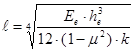Figure 118. Equation. Radius of relative stiffness.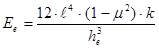Figure 119. Equation. Determination of elastic modulus.

Where:

Ee = Effective elastic modulus of the combined HMA and PCC layers, MPa (lbf/inch²).
ℓ = Radius of relative stiffness, mm (inches).
he = Effective thickness of the HMA and PCC layers, mm (inches).
μ = PCC Poisson’s ratio.
k = Modulus of subgrade reaction, MPa/mm (lbf/inch²/inch).

Note that the backcalculated radius of relative stiffness reflects the combined stiffness of both the HMA and PCC layers. The backcalculation of a two-layer plate on grade is based on the approach proposed by Ioannides and Khazanovich.(100) In this approach, the two layers are modeled as a single equivalent plate that has the same stiffness as the original system. The equivalent plate has the thickness equaling the combined thickness of the two layers, and the modulus is selected such that the equivalent plate has the same flexural stiffness as the original system. In this situation, either a fully bonded or fully unbonded interface can be modeled under the assumptions of plate theory; thus, no through-thickness compression is assumed. In the case of HMA/PCC pavements, the two surface layers are assumed to be bonded.

For two bonded layers, the following relationship (figure 120) exists between the equivalent plate and the two actual pavement layers:(100)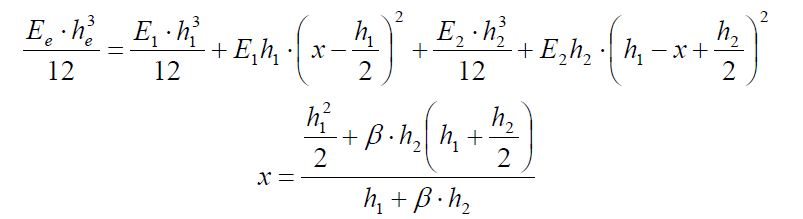Figure 120. Equation. Relationship between the equivalent plate and the pavement layers.(100)

Where:

Ee = Effective elastic modulus of the combined HMA and PCC layers, MPa (lbf/inch²).
he = Effective thickness of the HMA and PCC layers, mm (inches) = hHMA + hPCC.
E1 = Elastic modulus of the upper plate (HMA), MPa (lbf/inch²).
E2 = Elastic modulus of the lower plate (PCC), MPa (lbf/inch²).
h1 = Thickness of the upper plate (HMA), mm (inches).
h2 = Thickness of the lower plate (PCC), mm (inches).
x = Depth to the neutral axis, mm (inches).
β = Modular ratio = E2 /E1.

In the equation in figure 120, a similar Poisson’s ratio was assumed for the two bonded layers as a matter of convenience.

The moduli of the HMA (EAC) and PCC layers can be determined from the backcalculated Ee by rearranging the figure 120 equation and substituting β E1 for E2 and (h1+ h2) for he (figure 121).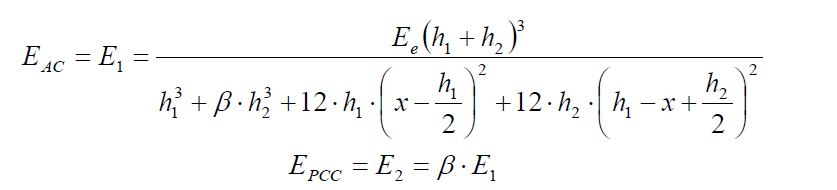Figure 121. Equation. Determination of moduli of the HMA and PCC layers.

One limitation of this approach is that the modular ratio β is unknown and must be assumed. However, as discussed in chapter 5, the backcalculated PCC modulus is relatively insensitive to the assumed value of β for a fairly wide range of values. This is illustrated in figure 122. However, the backcalculated HMA modulus could be off significantly, if the assumed value of β is incorrect.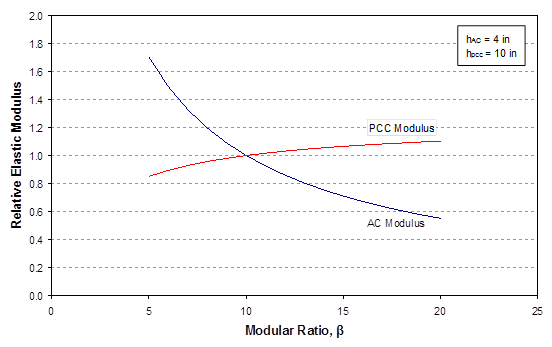1 inch = 25.4 mm.

Figure 122. Graph. Sensitivity of the backcalculated moduli values to the assumed value of the modular ratio β.(100)

For the evaluation of the structural adequacy of the PCC layer, only the overall stiffness of the pavement layers and PCC modulus are significant; the HMA modulus does not directly affect the PCC stress calculation. In fact, for a composite pavement, the modulus of the existing PCC layer is the only bound layer for which backcalculated data are used as an input in the MEPDG.(7)

Best-Fit Method

In this approach, the modulus of the pavement layers and subgrade k-value are estimated by finding the combination that provides the best match between the calculated and measured deflections. As discussed in chapter 5, the problem is formulated as minimization of the error function, F, as shown in figure 123.(51)Figure 123. Equation. Minimization of the error function, F.(51)

Where:

E = Effective elastic modulus of the combined HMA and PCC layers, MPa (lbf/inch²).
k = Modulus of subgrade reaction, MPa/mm (lbf/inch²/inch).
αi = Weighting factors.
wi = Calculated deflection at sensor location i, mm (inches).
Wi = Measured deflection at sensor location i, mm (inches).

The weighting factors could be set equal to 1, a function of measured deflection (Wi), or any other number. For the case of composite pavements, setting α0 equal to 0 for the d0 deflection and αi equal to 1 for the remaining sensors gives equal weight to the sensors used and excludes the d0 deflection from the calculation. The details of the Best-Fit method are given in chapter 5.

The radius of relative stiffness backcalculated by this approach again reflects the combined stiffness of the HMA and PCC layers. The same steps described for the outer-AREA method must be followed to obtain EHMA and EPCC from the combined pavement layer stiffness, based on the assumed value of the modular ratio β. The Best-Fit method was determined to provide more consistent results and recommended for use in an LTPP data analysis study.(51)

### APPLICABILITY FOR MEPDG USE

The MEPDG evaluates HMA/PCC pavements in two steps. First, the pavement system is analyzed as a rigid pavement to model continued cracking of the underlying PCC pavement. The HMA distresses are then modeled, including thermal cracking, fatigue cracking, and rutting, as well as the International Roughness Index. Assuming that the analysis is to be conducted for a new HMA overlay, the key input parameters for this analysis obtained from FWD data are the subgrade k-value, EPCC, and PCC modulus of rupture. Although, the PCC modulus of rupture can be estimated from backcalculated EPCC using an empirical correlation, limited core testing is highly recommended to verify the values.(103) The joint LTE is also used in reflection cracking prediction, but only qualitative results are used (good or poor).

The backcalculation results for HMA/PCC pavements may contain greater variability than those for other pavement types, largely because the data may contain the results for tests conducted over joints or cracks in the underlying PCC pavement. For valid results, the locations of the joints in the underlying pavement should be identified and the testing conducted should be performed at midslab. Any significant deviations from the representative values may be an indication that the testing was conducted too close to underlying cracks or joints, and those results should be excluded in determining the average k-value and average E. For the evaluation of the structural adequacy of the underlying PCC pavement, the elastic modulus determined over the intact portion of the slab is needed.

For rehabilitation design, the MEPDG allows the dynamic backcalculated k-value to be entered directly.(7) Both the representative k-value and month of testing are entered. However, the backcalculated k-value is an optional input; the user is still required to enter resilient moduli for all unbound layers and subgrade. The MEPDG processes the input as usual (for new design) and determines the seasonal k-values based on EICM results and using the E-to-k conversion procedure. If the backcalculated k-value is entered, the seasonal adjustment is made using the relative k-values obtained through the E-to-k conversion process as the scaling factors.

For the HMA analysis, the seasonal resilient moduli are used, but no adjustment is made to account for any difference between the k-value from the E-to-k conversion process and the backcalculated k-value. To ensure consistency between the backcalculated k-value and the resilient moduli used in the HMA analyses, an iterative procedure may be used to adjust the subgrade resilient modulus input, rather than simply entering the backcalculated k-value. This involves adjusting the input subgrade resilient modulus up or down to match the k-value from the E-to-k conversion process and the backcalculated k-value for the month of the FWD testing. If the backcalculated k-value is entered directly, there may be some discrepancy between the k‑value used in the PCC analysis and the resilient moduli used in the HMA analysis; however, this minor discrepancy is not likely to have any significant effect on the predicted HMA overlay performance.

### RECOMMENDATIONS FOR MEPDG USE

The evaluation of FWD data is perhaps more difficult for HMA/PCC pavements than for other pavement types because of the complications introduced by the compression of the HMA layer. On the other hand, the structural adequacy is not always the principal concern for HMA/PCC pavements. Placing even a relatively thin layer of HMA (75 to 100 mm (3 to 4 inches)) has the effect of reducing the critical stresses in the underlying PCC pavement, because of the following:

• Additional stiffness provided by the HMA overlay. The HMA overlay bonds to the PCC layer to provide a significant increase in the structural capacity.

• Significant reduction in temperature gradients. On PCC pavements, the curling stresses can make up more than 50 percent of the combined stresses during the critical periods, when the pavement is subjected to high temperature gradients (positive or negative). The most severe temperature changes occur in the top 75 mm (3 inches) of the pavement. Placement of an HMA overlay significantly reduces the magnitude of the temperature gradients.

• Reduction in contact pressure. The HMA layer reduces the contact pressure on PCC pavement by spreading the load over a larger area.

The combined effect of these factors is that the critical stresses in the PCC pavement drop to a fraction of those prior to overlaying, even for a minimum-thickness HMA overlay (e.g., 75 mm (3 inches)). Thus, the performance life of HMA/PCC is typically governed by material and functional factors, not structural failure of the underlying PCC pavement. The key distresses include rutting, reflection cracking, and deterioration of the reflected cracks. The structural evaluation of HMA/PCC pavements is mainly a design check to ensure that the stresses are well within the tolerable limits. Therefore, the backcalculation procedure for HMA/PCC does not need to be as comprehensive.

Among the backcalculation procedures reviewed (including the most recent ANN-based procedures), the convenience and accessibility of the outer-AREA method outweigh the benefits of any advantages in accuracy the other methods may offer. For HMA overlay thicknesses up to about 254 mm (10 inches), the backcalculated k-values obtained using the outer-AREA method closely match those obtained by other, more sophisticated methods. For the backcalculated elastic moduli values, the ANN-based method offers a slight advantage in accuracy, and that approach does not involve estimating the modular ratio β (EPCC/EHMA) in advance.(109) For the backcalculated moduli, the Best-Fit method does not offer a significant advantage over the outer-AREA method. Both methods involve estimating the modular ratio β to resolve the backcalculated effective E into component moduli values; therefore, the slight advantage of the Best-Fit method in accuracy is not very meaningful.

One issue not previously discussed is the handling of the presence of a stabilized base under the PCC pavement. For the HMA surface layer, the compression of the HMA actually provides additional information that could be used to determine the HMA modulus, if a structural model that considers through-thickness compression were used (e.g., an elastic layer program). However, for the stabilized layers beneath the PCC layer, there may be no way to precisely determine the layer modulus from the surface deflection data. Given that the bending stiffness of multiple pavement layers (plates) can be represented by an equivalent plate with an effective thickness (he) and modulus (Ee), it is not possible to resolve the backcalculated effective modulus into component moduli without having additional information, namely the modular ratio and interface bonding condition. Because these ratios can only be estimated, the precise determination of individual layer moduli is not possible. This may be an inherent limitation of the backcalculation process for composite pavements, but because PCC pavement properties are relatively consistent over the length of a project, a limited amount of core testing can be conducted to obtain the additional information needed to verify backcalculation results.

In light of the discussion above, the following steps are recommended for the backcalculation of HMA/PCC pavements:

• Use the outer-AREA method.

• Conduct limited core testing to verify backcalculation results and the modular ratios assumed for backcalculation.

As shown in figure 122, the backcalculated EPCC is not highly sensitive to the modular ratio β over a relatively wide range of β values. The following empirical correlation between HMA modulus and temperature and typical PCC moduli for the region may be used to obtain a good estimate of β (figure 124):(111)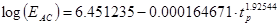Figure 124. Equation. Empirical correlation between HMA modulus and temperature.(111)

Where:

EHMA = Elastic modulus of HMA lbf/inch²/inch.
tp = HMA mix temperature °F.

The slab size correction need not be made for backcalculation results of HMA/PCC pavements. William showed that the deflection behavior of doweled pavements is similar to that of the infinite slabs assumed in the structural models used in backcalculation.(113) For HMA/PCC pavements, the HMA overlay contributes to the continuity of the pavement structure across transverse joints. Thus, no correction for the slab size is recommended for the backcalculation results for HMA/PCC pavements.

### FUTURE RESEARCH NEEDS

Although the available analysis tools are lacking in their ability to fully model the behavior of HMA/PCC pavements, this limitation does not seriously impair the ability to evaluate FWD data for HMA/PCC pavements. That is, the limitations of the available tools do not have a significant practical impact on the ability to evaluate the characteristics of HMA/PCC pavements. The subgrade k-value can be determined reliably using the simplest of the available tools, the outer-AREA method. Reasonable estimates can also be made for the PCC elastic modulus using the outer-AREA method. As with the other pavement types, a limited amount of core testing is highly recommended to evaluate the material properties and to validate the pavement layer moduli determined from FWD data. The typical reasons for rehabilitating HMA/PCC pavements are commonly related to material and functional issues. The continued deterioration of the underlying PCC pavement is rarely the principal mode of deterioration for HMA/PCC pavements. The results obtained using the available analysis tools are adequate for the purposes of conducting what is essentially a design check on rehabilitation design of HMA/PCC pavements.

Nevertheless, improved models for both forward analysis and backcalculation would help more accurately model the behavior of composite pavements. The structural models based on plate theory do not correctly model the behavior of HMA/PCC pavements because the through-thickness deformations are ignored. Elastic layer programs do not yield the k-value typically needed for the analysis of PCC pavements. An elastic layer program that allows the subgrade to be modeled as a Winkler foundation has been developed, which may be ideal for the backcalculation of HMA/PCC pavements.(109) Researchers have also demonstrated the advantages of using ANNs for backcalculation. Development of software specifically designed for the backcalculation of HMA/PCC pavements (complete with an automated process for data screening to identify problem data points) would facilitate the process of analyzing the FWD data.

Federal Highway Administration | 1200 New Jersey Avenue, SE | Washington, DC 20590 | 202-366-4000
Turner-Fairbank Highway Research Center | 6300 Georgetown Pike | McLean, VA | 22101